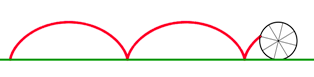# CycloidAllPages RecentChanges Links to this page Edit this page Search Entry portal Advice For New Users

The cycloid is a plane curve. The cycloid is the locus of a point on the rim of a circle rolling along a straight line.An animation of the generation of a cycloid can be found at http://mathworld.wolfram.com/Cycloid.html

The parametric equations of a cycloid passing through the origin generated by a circle of radius a in Cartesian coordinates are

• $x=a(t-\sin~t)$
• $y=a(1-\cos~t)$
http://en.wikipedia.org/wiki/Cycloid

The cycloid is the solution of the Brachistochrone Problem - Find the shape of the curve in which a wire must be made so that a bead will slide freely under gravity from one point to another in the same vertical plane in the shortest time.

Therefore, if a ski resort wanted to get skiers from the top to the bottom of a mountain in the shortest time, the mountain should carved out into the shape of a cycloid.

The cycloid is also the solution of the Tautochrone problem - Find the shape of the curve in which a wire must be made so that the time taken by a bead sliding without friction under gravity to its lowest point is independent of its starting point.

A pendulum does not keep perfect time if the amplitude (angle) of swing changes. However if a bead is allowed to swing on a frictionless wire in the shape of a cycloid it will keep the same time whatever the size of the oscillation.

Christian Huygens was able to use this property of the cycloid to make an improvement to the simple pendulum clock.

One of the named curves on this site